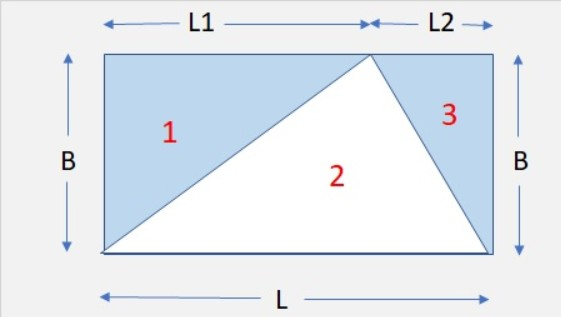# QuestionArea of rectangle -> B × L = BL

Area of unshaded triangle 2 -> ½ × B × L = ½BL

Area of shaded parts -> area of rectangle – area of unshaded triangle 2 = BL – ½BL = ½BL

Alternatively

Area of triangle 1 -> ½ × B × L1 = ½BL1

Area of triangle 3 -> ½ × B × L2 = ½BL2

Area of shaded parts -> ½BL1 + ½BL2 = ½B(L1 + L2)

But since L1 + L2 = L, area of shaded parts -> ½BL

½BL is half of the rectangle

(a)
area of triangle ABQ = (1/2) x (5 + 5) x 5 = 25 sq cm

(b)
area of triangle BPQ = area of triangle ABQ = 25 sq cm
area of BCQ = (1/2) x 10 x 5 = 25 sq cm
total area of shaded part = 25 + 25 + [(1/2) x 3.14 x 5 x 5 – 25] = 64.25 sq cm

Ans : (a) 25 sq cm; (b) 64.25 sq cm.

0 Replies 0 Likes

Oh, I just realised that half of the rectangle is also shaded.

Area of triangle PBQ = 0.5 × 10 × 5 = 25

Area of triangle BCQ = Area of triangle ABQ = 25

Area of triangle PCQ = 25 + 25 = 50

Total area of the shaded parts = 14.25 + 50 = 64.25 cm²Area of rectangle -> B × L = BL

Area of unshaded triangle 2 -> ½ × B × L = ½BL

Area of shaded parts -> area of rectangle – area of unshaded triangle 2 = BL – ½BL = ½BL

Alternatively

Area of triangle 1 -> ½ × B × L1 = ½BL1

Area of triangle 3 -> ½ × B × L2 = ½BL2

Area of shaded parts -> ½BL1 + ½BL2 = ½B(L1 + L2)

But since L1 + L2 = L, area of shaded parts -> ½BL

½BL is half of the rectangle